Plot the columns sp and/or elev of ForestGEO-like datasets of class 'sp' and/or 'sp_elev'.

• You can create a 'sp' object with:

    object <- sp(DATA-WITH-VARIABLE-sp)

• You can create an 'elev' object with:

    object <- elev(DATA-WITH-VARIABLE-elev)

• You can create a 'sp_elev' object with:

    object <- sp_elev(DATA-WITH-VARIABLE-sp, DATA-WITH-VARIABLE-elev)


See Examples below.

# S3 method for sp_elev
autoplot(
object,
fill = "sp",
hide_fill_legend = FALSE,
shape = 21,
point_size = 3,
facet = TRUE,
contour_size = 0.5,
low = "blue",
high = "red",
hide_color_legend = FALSE,
bins = NULL,
label_size = 3,
label_color = "grey",
xyjust = 1,
fontface = "italic",
xlim = NULL,
ylim = NULL,
custom_theme = NULL,
...
)

# S3 method for sp
autoplot(
object,
fill = "sp",
hide_fill_legend = FALSE,
shape = 21,
point_size = 3,
facet = TRUE,
xlim = NULL,
ylim = NULL,
custom_theme = NULL,
...
)

# S3 method for elev
autoplot(
object,
contour_size = 0.5,
low = "blue",
high = "red",
hide_color_legend = FALSE,
bins = NULL,
label_size = 3,
label_color = "grey",
xyjust = 1,
fontface = "italic",
xlim = NULL,
ylim = NULL,
custom_theme = NULL,
...
)

## Arguments

object An object created with sp(), elev(), or sp_elev(). Character; either a color or "sp", which maps each species to a different color. Logical; TRUE hides the fill legend. A number giving point shape (as in graphics::points()). Passed to ggplot2::geom_point(). A number giving point size. Passed to ggplot2::geom_point(). Logical; TRUE wraps multiple panels within the area of a single graphic plot. A number giving the size of the contour of elevation lines. Passed to ggplot2::stat_contour() (see ggplot2::geom_contour()). A string giving a color of the elevation lines representing low and high elevation. Logical; TRUE hides the color legend. A number giving the number of elevation lines to plot. Logical; FALSE hides elevation labels. A number (label_size) or character string (label_color and fontface) giving the size, colour and fontface of the text labels for the elevation lines. A number to adjust the position of the text labels of the elevation lines. A vector of length 2, for example c(0, 500), giving the minimum and maximum limits of the vertical and horizontal coordinates. A valid ggplot2::theme(). NULL uses the default theme theme_default(). Not used (included for compatibility across methods).

A "ggplot".

## Details

autoplot(sp_elev(DATA-WITH-VARIABLE-sp) (without elevation data) is equivalent to autoplot(sp(DATA-WITH-VARIABLE-sp)).

fgeo.plot wraps some functions from the ggplot2 package. For more control you can use ggplot2 directly.

Other plot functions: autoplot.fgeo_habitat(), autoplot_by_species.sp_elev(), elev(), plot_dbh_bubbles_by_quadrat(), plot_tag_status_by_subquadrat(), sp_elev(), sp()

Other autoplots: autoplot.fgeo_habitat(), elev(), sp_elev(), sp()

Other functions to plot elevation: autoplot_by_species.sp_elev(), elev(), sp_elev()

Other functions to plot species: autoplot_by_species.sp_elev(), sp_elev(), sp()

## Examples

assert_is_installed("fgeo.x")

# Species ---------------------------------------------------------------

# Small dataset with a few species for quick examples
census <- fgeo.x::tree5 %>%
subset(sp %in% c("PREMON", "CASARB"))

autoplot(sp(census))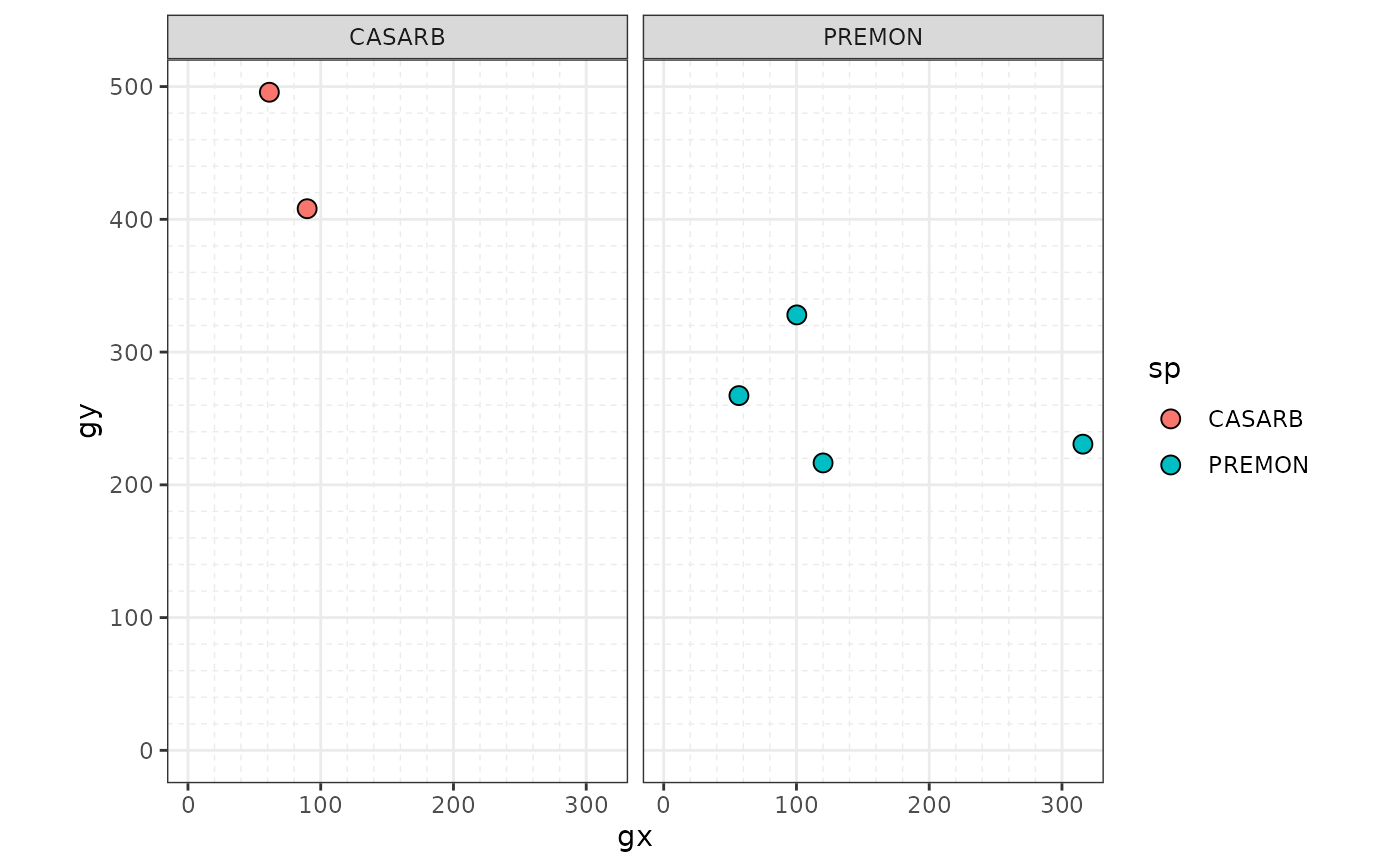# Skip R CMD check for speed
# \donttest{
# Customize
autoplot(sp(census), point_size = 1)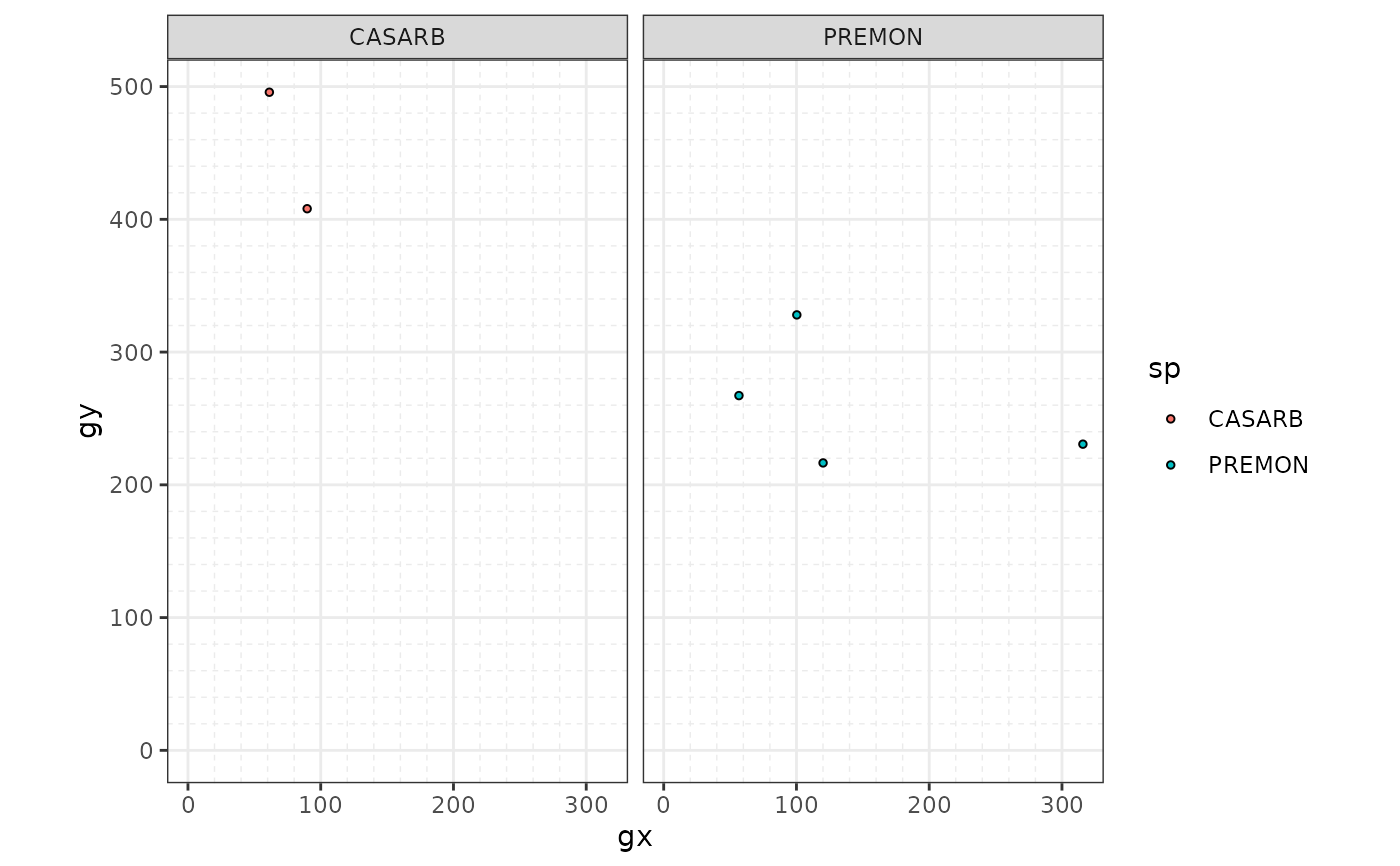# Elevation -------------------------------------------------------------

elevation <- fgeo.x::elevation
autoplot(elev(elevation))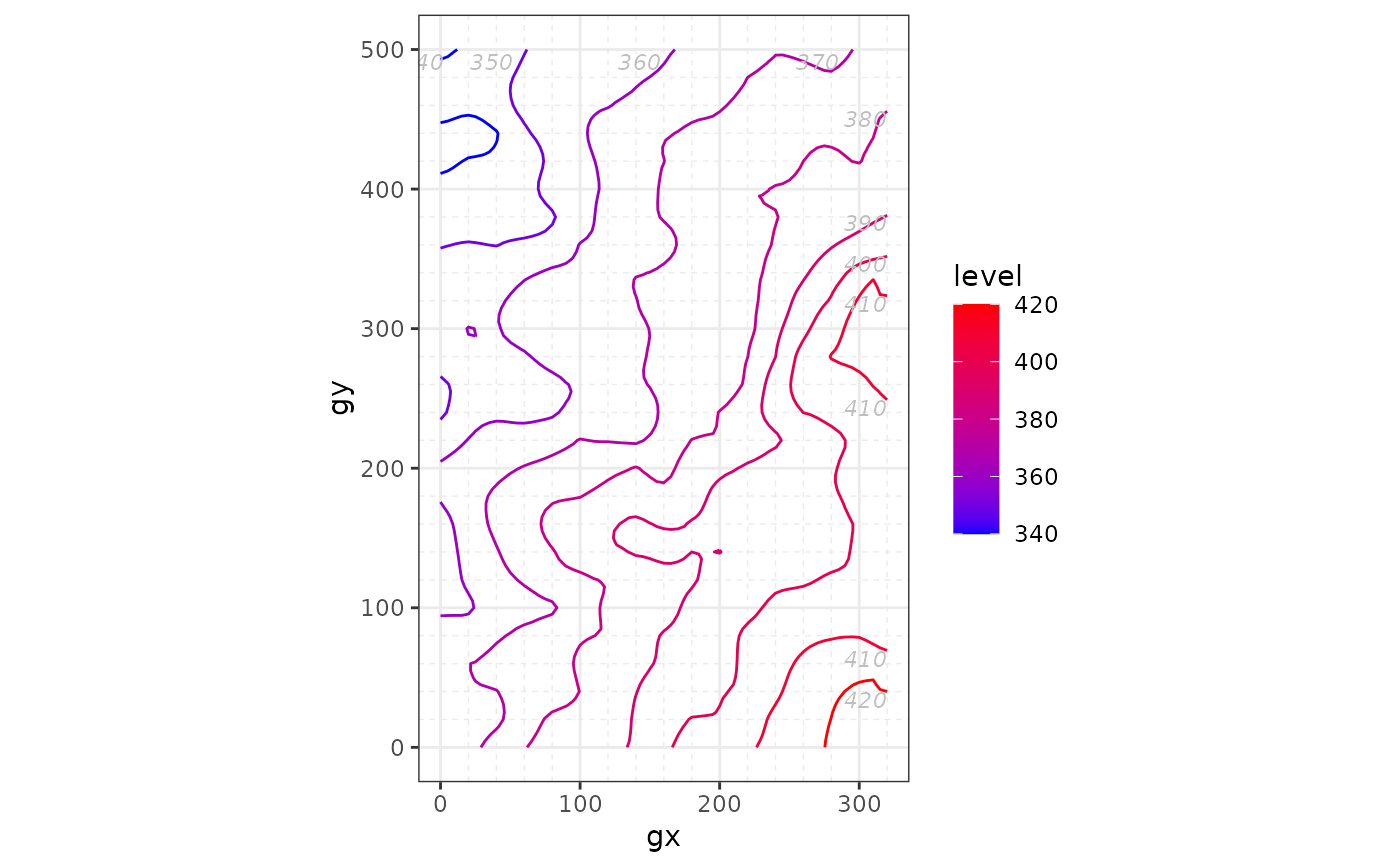# Skip R CMD check for speed
# Same as autoplot(elev(elevation))
autoplot(elev(elevation\$col))# Customize
autoplot(elev(elevation), contour_size = 1)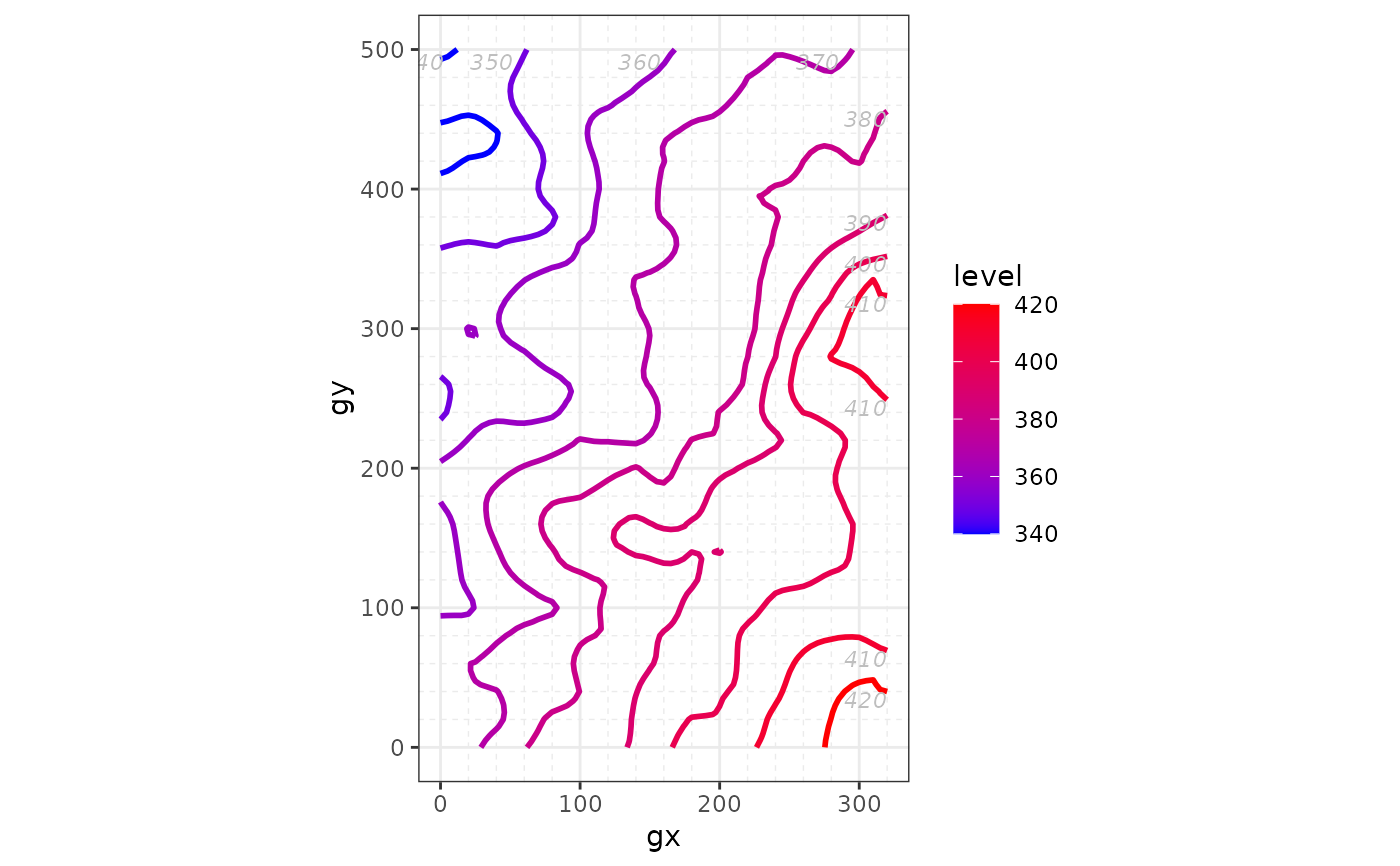# Species and elevation -------------------------------------------------
autoplot(sp_elev(census, elevation), facet = FALSE, point_size = 1)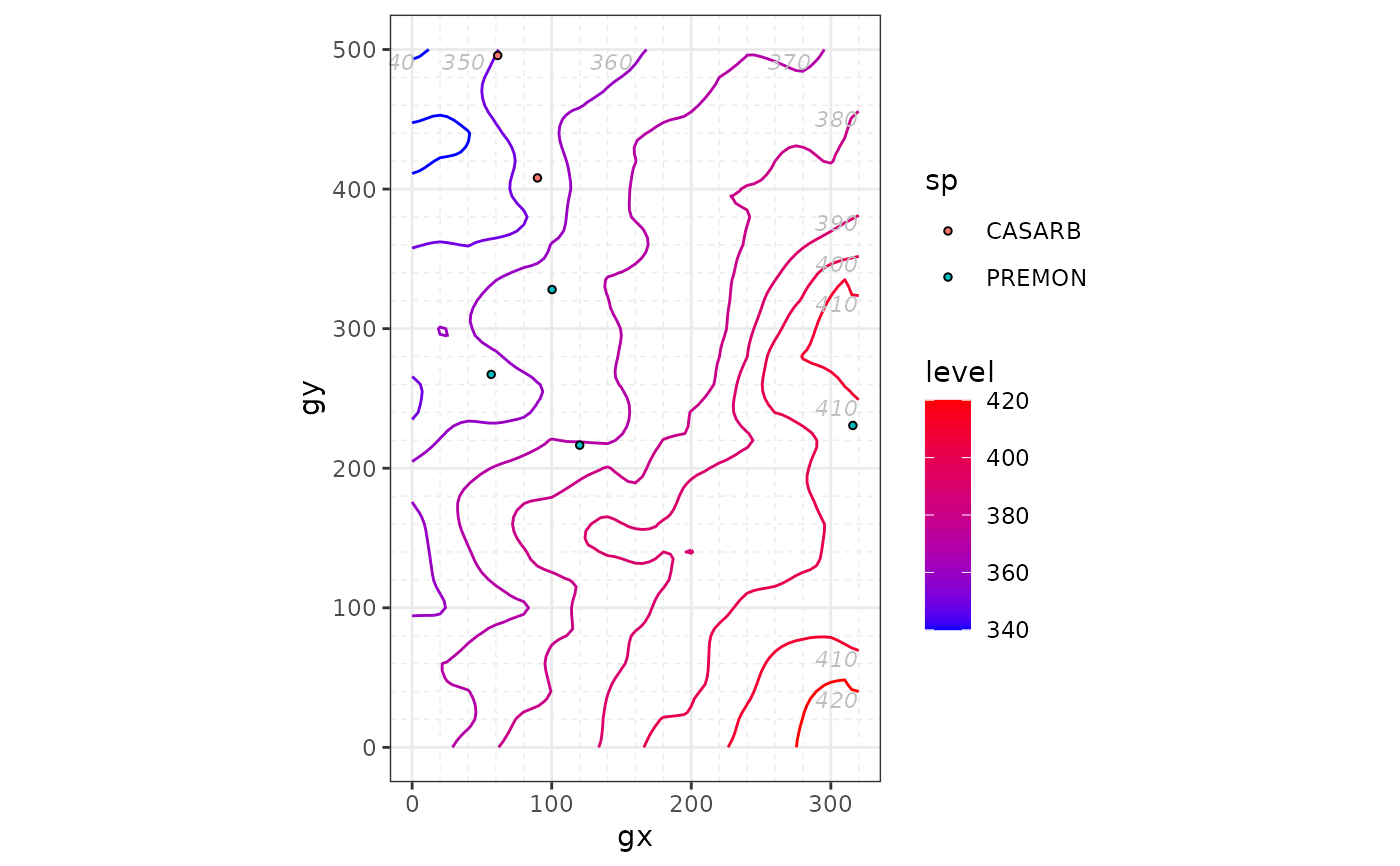# }